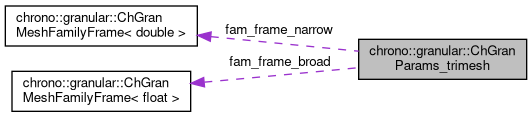chrono::granular::ChGranParams_trimesh Struct Reference

## Description

Extra parameters needed for triangle-sphere contact.

#include <ChGranularTriMesh.h>

Collaboration diagram for chrono::granular::ChGranParams_trimesh:[legend]

## Public Attributes

float K_n_s2m_SU
Sphere-to-mesh normal stiffness, expressed in SU (Hertzian spring)

float K_t_s2m_SU
Sphere-to-mesh tangent stiffness, expressed in SU (Hertzian spring)

float Gamma_n_s2m_SU
Sphere-to-mesh normal contact damping coefficient, expressed in SU.

float Gamma_t_s2m_SU
Sphere-to-mesh tangent contact damping coefficient, expressed in SU.

float static_friction_coeff_s2m
Ratio of normal force to peak tangent force, also arctan(theta) where theta is the friction angle.

float rolling_coeff_s2m_SU
Coefficient of rolling resistance. Units and effect depend on the system rolling resistance model.

float spinning_coeff_s2m_SU
Coefficient of spinning resistance. Units and effect depend on the system spinning resistance model.

unsigned int num_triangle_families
Number of triangle families.